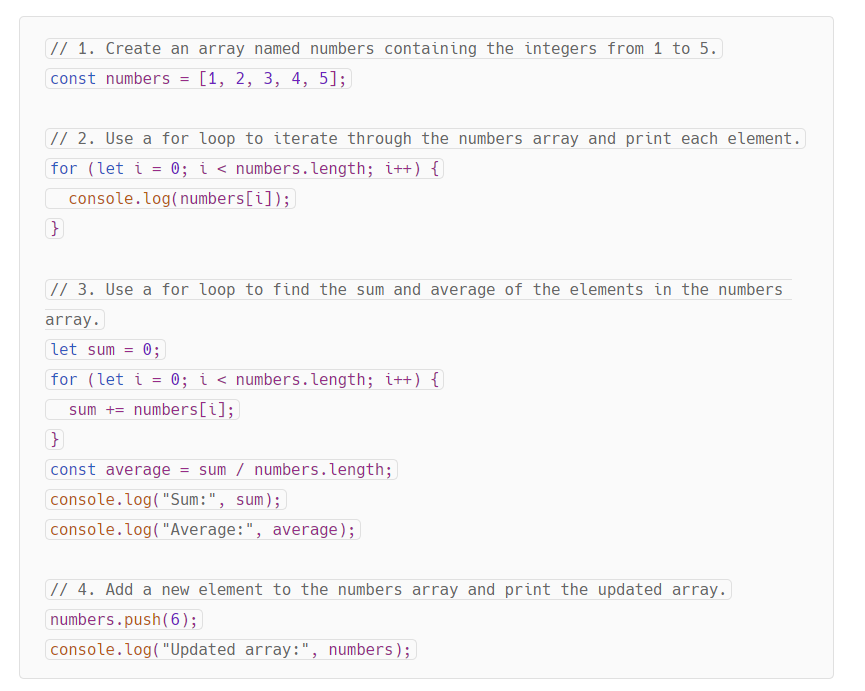Share
Explore# JavaScript Student Lab Exercises Workbook

Welcome to the JavaScript Student Lab Exercises Workbook! This workbook contains 10 progressively more complex exercises designed to help you learn and practice JavaScript fundamentals, such as variables, loops, if-then statements, functions, and input-output operations.

Exercise 1: Variables and Basic Arithmetic
Exercise 2: User Input and Output
Exercise 3: If-Then Statements
Exercise 4: For Loops
Exercise 5: While Loops
Exercise 6: Functions
Exercise 7: More Complex Functions
Exercise 8: Arrays
Exercise 9: Objects
Exercise 10: DOM Manipulation

## Exercise 1: Variables and Basic Arithmetic

Objective: Create variables, perform basic arithmetic operations, and display the results.
Declare a variable named num1 and assign it the value 10.
Declare a variable named num2 and assign it the value 20.
Calculate the sum, difference, product, and quotient of num1 and num2, storing the results in variables named sum, difference, product, and quotient, respectively.
Print the values of sum, difference, product, and quotient.

## Exercise 2: User Input and Output

Objective: Accept user input, perform calculations, and display output.
Ask the user to enter two numbers.
Store the user input in variables named input1 and input2.
Convert the user input into numbers, and store the result in variables named number1 and number2.
Calculate the sum of number1 and number2, and store the result in a variable named total.
Display the value of total to the user.

## Exercise 3: If-Then Statements

Objective: Implement a basic if-then statement to compare two numbers.
Ask the user to enter two numbers.
Store the user input in variables named num1 and num2.
Compare the two numbers using an if-then statement.
Display the result of the comparison (e.g., "Number 1 is greater than Number 2").

## Exercise 4: For Loops

Objective: Implement a basic for loop to perform repetitive tasks.
Declare a variable named iterations and assign it the value 5.
Use a for loop to print the numbers from 1 to iterations.
Modify the for loop to print the square of each number from 1 to iterations.

## Exercise 5: While Loops

Objective: Implement a basic while loop to perform repetitive tasks.
Declare a variable named counter and assign it the value 1.
Use a while loop to print the numbers from 1 to 5.
Modify the while loop to print the cube of each number from 1 to 5.

## Exercise 6: Functions

Objective: Create and use basic functions.
Create a function named add that takes two parameters, a and b, and returns their sum.
Create a function named subtract that takes two parameters, a and b, and returns their difference.
Create a function named multiply that takes two parameters, a and b, and returns their product.
Create a function named divide that takes two parameters, a and b, and returns their quotient.
Call each of these functions with sample inputs and display the results.

## Exercise 7: More Complex Functions

Objective: Create more complex functions that involve loops and conditional statements.
Create a function named factorial that takes a parameter num and returns its factorial.
Create a function named isPrime that takes a parameter num and returns true if the number is prime, false otherwise.
Create a function named fibonacci that takes a parameter n and returns the nth Fibonacci number.
Call each of these functions with sample inputs and display the results.

## Exercise 8: Arrays

Objective: Work with arrays to store and manipulate data.
Create an array named numbers containing the integers from 1 to 5.
Use a for loop to iterate through the numbers array and print each element.
Use a for loop to find the sum and average of the elements in the numbers array.
Add a new element to the numbers array and print the updated array.
Here's the complete code for Exercise 8 in JavaScript:
`⁠⁠⁠`
This code creates an array of integers from 1 to 5, iterates through the array to print each element, finds the sum and average of the elements, and adds a new element to the array.

## Exercise 9: Objects

Objective: Create and manipulate objects in JavaScript.
Create an object named person with properties firstName, lastName, age, and city.
Print the firstName and lastName properties of the person object.
Add a new property named country to the person object, and set its value.
Print the updated person object.
Here's a step-by-step guide to create and manipulate objects in JavaScript, based on the objectives provided:

### Exercise 1: Create an object named person with properties firstName, lastName, age, and city.

`javascript``Copy code``let person = {`` firstName: "John",`` lastName: "Doe",`` age: 30,`` city: "New York"``};`

### Exercise 2: Print the firstName and lastName properties of the person object.

`javascript``Copy code``console.log("First Name:", person.firstName);``console.log("Last Name:", person.lastName);`

### Exercise 3: Add a new property named country to the person object, and set its value.

`javascript``Copy code``person.country = "USA";`

### Exercise 4: Print the updated person object.

`javascript``Copy code``console.log(person);`
Now let's put it all together in a single script:
```// Exercise 1: Create an object named person with properties firstName, lastName, age, and city. let person = { firstName: "John", lastName: "Doe", age: 30, city: "New York" };``````// Exercise 2: Print the firstName and lastName properties of the person object. console.log("First Name:", person.firstName); console.log("Last Name:", person.lastName);``````// Exercise 3: Add a new property named country to the person object, and set its value. person.country = "USA";``````// Exercise 4: Print the updated person object. console.log(person);```

## Exercise 10: DOM Manipulation

Objective: Learn how to manipulate the DOM using JavaScript.

Create an HTML file with a div element having an id attribute with the value "content".

Create a JavaScript file and link it to the HTML file.